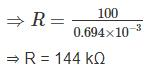Courses

# Test: Electrical And Electronic Measurements- 2

## 10 Questions MCQ Test RRB JE for Electrical Engineering | Test: Electrical And Electronic Measurements- 2

Description
This mock test of Test: Electrical And Electronic Measurements- 2 for Electrical Engineering (EE) helps you for every Electrical Engineering (EE) entrance exam. This contains 10 Multiple Choice Questions for Electrical Engineering (EE) Test: Electrical And Electronic Measurements- 2 (mcq) to study with solutions a complete question bank. The solved questions answers in this Test: Electrical And Electronic Measurements- 2 quiz give you a good mix of easy questions and tough questions. Electrical Engineering (EE) students definitely take this Test: Electrical And Electronic Measurements- 2 exercise for a better result in the exam. You can find other Test: Electrical And Electronic Measurements- 2 extra questions, long questions & short questions for Electrical Engineering (EE) on EduRev as well by searching above.
QUESTION: 1

### An ideal diode has been connected across a 10 Ω, 100 mA, center-zero PMMC meter as shown in figure. The meter will read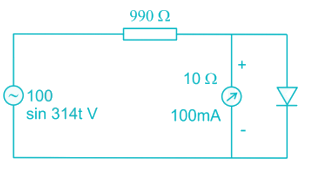Solution:

In positive half cycle, the diode gets forward biased. Hence diode is in on state so, no current will flows through ammeter.
In negative half cycle, the diode gets reverse biased. Hence diode is in off state. So, the current flows through ammeter.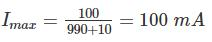The current waveform will be,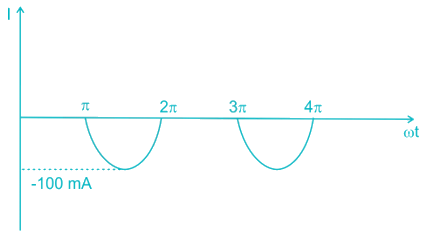PMMC instrument measures average value.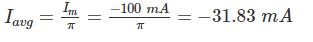*Answer can only contain numeric values
QUESTION: 2

### The deflecting torques of an ammeter varies as the square of the current passing through it. If a current of 15A produces a deflection of 30°, The deflection of a current of 20 A when instrument is spring controlled will be _____________ (in degrees).

Solution:

Td = KdI2
for spring control Tc = Kcθ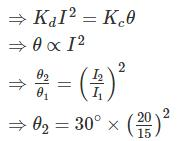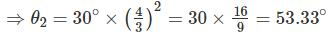QUESTION: 3

### A moving iron instrument has 10 Ω resistance and gives full scale deflection when carrying 50 mA. It can be used measure 750 V by using what resistance?

Solution:

Given that,
Full scale deflection = 50 mA
Internal resistance of instrument = 10 Ω
We can increase the range of voltage by using series resistance.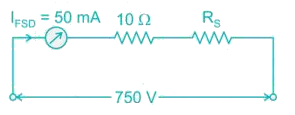750 = (10 + RS) × 50 × 10-3
⇒ RS + 10 = 15,000
⇒ RS = 14990 Ω
Hence the resistance should be connected in series.

*Answer can only contain numeric values
QUESTION: 4

What will be the reading (in V) when a voltage e = 200 sin ωt + 40 sin (3ωt + 30°) + 30 cos (5 ωt + 21.5°) v is applied to an electrodynamometer voltmeter.

Solution:

Electrodynamometer voltmeter reads the rms value.
Given that,
e = 200 sin ωt + 40 sin (3ωt + 30°) + 30 cos (5 ωt + 21.5°) V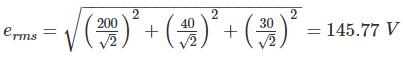QUESTION: 5

A 600 kΩ resistor is connected from the 200 V terminal to the common terminal of a dual scale voltmeter, as shown in figure (a). This modified voltmeter is then used to measure the voltage across the 360 kΩ resistor in the circuit in figure (b). What is the reading on the 500 V scale of the meter?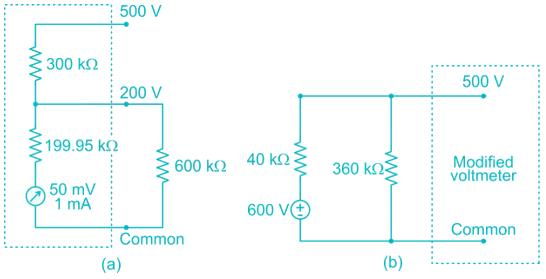Solution:

From the figure (a)
Internal resistance of galvanometer = 50 mV/1 mA
= 50 Ω
Now the circuit becomes,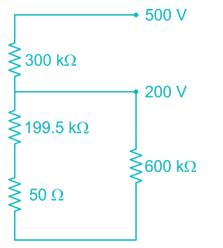Total internal resistance of voltmeter for 500 V scale,
Rm = 300 + [(199.5 + 0.05)||600]
= 300 + [200||600]
= 300 + 150 = 450 kΩ
Now, the voltmeter with internal resistance of 450 kΩ and 500 V scale is used to measure voltage in the circuit shown in fig (b).
Now, the circuit becomes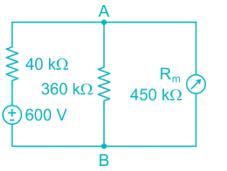Because of voltmeter resistance, the load resistance becomes = 360||450 = 200 kΩ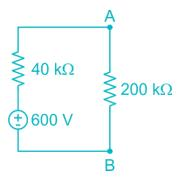By voltage division,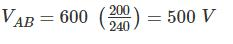*Answer can only contain numeric values
QUESTION: 6

The spring constant of a 3000 V electrostatic voltmeter is 7.06 × 10-6 Nm/rad. The full scale deflection of the instrument is 80°. Assuming the rate of change of capacitance with angular deflection to be constant over the operating range. Calculate the total change of capacitance (in pF) from zero to full scale.

Solution:

We know that,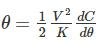Given that,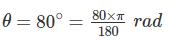V = 3000 V
K = 7.06 × 10-6 Nm/rad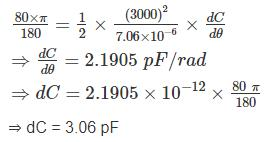QUESTION: 7

Two different a.c, voltmeters are used to measure the voltage across the 22 kΩ resister shown in figure. Meter A has a.c. sensitivity of 10 kΩ/V, a guaranteed accuracy of 98% at full scale, and is set on its 200V scale. Meter B has an a.c. sensitivity of 4kΩ/V. a guaranteed accuracy of 98.5% at full scale, and is set on its (0-100)V range. Which meter Provides more accurate results?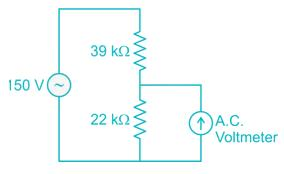Solution:

Sensitivity of meter A, SA = 10 kΩ/V
Full scale reading of meter A, VFSD(A) = 200 V
Internal resistance of meter A,
RV(A) = 10 kΩ/V × 200 V
= 2000 KΩ
Guaranteed accuracy of Meter A = 98% at FSD.
Error of meter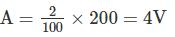Sensitivity of meter B, SB = 4KΩ/V
Full Scale reading of meter B, VFSD = 100V
Internal resistance of meter B,
RV(B) = 4 KΩ/V × 100 = 400 KΩ
Guaranteed accuracy of meter B = 98.5% at FSD
Error of meter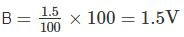True reading across 22 KΩ resister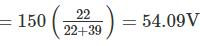If we use meter A, then the reading will be the effective will be, The effective resistance across 22kΩ
= 22k ||2000k = 21.76 kΩ
Now the reading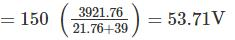But the meter has an error of 4V
Hence the range of reading = [49.71, 57.71]
If we use meter B, then the reading will be
The effective resistance across 22kΩ,
= 22k||400k = 20.85kΩ
Now, the reading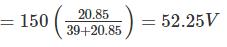But the meter has an error of 1.5V
Hence, the range of reading = [50.75, 53.75]
By observing both the readings we can conclude that meter B provides accurate results.

*Answer can only contain numeric values
QUESTION: 8

A moving iron instrument is calibrated with full scale deflection on 100 V DC. This instrument is used to measure 100 V at 50 Hz supply. Find the percentage error in reading if the instrument has a resistance of 2 kΩ and an inductance of 0.5 H

Solution:

Full scale deflection of instrument (VFSD) = 100V
Resistance (Rm) = 2 kΩ
Inductance (Lm) = 0.5 H
XL = 2πfL = 2π × 50 × 0.5 = 157.08 Ω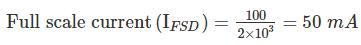Current through meter when 100 V at 50 Hz supply is applied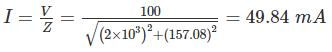Voltage reading of meter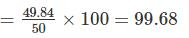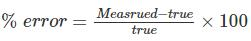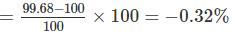*Answer can only contain numeric values
QUESTION: 9

An input voltage represented by wave from as shown below is applied to a PMMC instrument. The reading of the instrument will be __ ( in V)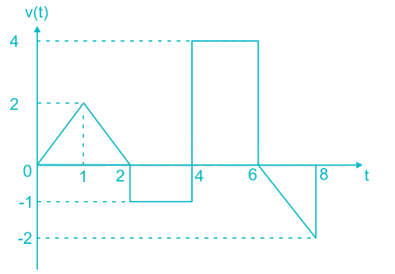Solution:

PMMC measures average value.
The average value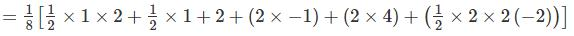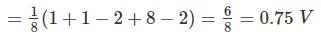QUESTION: 10

The coil of a moving coil voltmeter is 50 mm long and 40 mm wide and has 120 turns on it. The control spring exerts a torque 200 × 10-6 n – m when the deflection is 100 divisions on full scale. If the flux density of the magnetic field in the air gap is 1.2 wb/m2. The resistance that must be put in series with the coil to give one volt per division.

Solution:

Tc = 200 × 10-6 N – m
Td = B I N A
= 1.2 × I × 120 × 50 × 40 × 10-6
= 288 × 10-3 I n – m
At equilibrium,
Tc = Td
⇒ 200 × 10-6 = 288 × 10-3 I
⇒ I = 0.694 mA.
Let the resistance of the voltmeter circuit be R voltage across the instrument = 0.694 × 10-3 R
This produces a deflection of 100 divisions.
Volts per division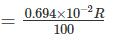This value should be equal to 1 in order to get 1 volt per division.